Номер части: Журнал
ISSN: 2411-6467 (Print)
ISSN: 2413-9335 (Online)
Статьи, опубликованные в журнале, представляется читателям на условиях свободной лицензии CC BY-ND

# DECISION — MAKING SCHEME FOR ENSEMBLE OF NEURAL NETWORKS IN TASK OF INTELLECTUAL DATA ANALYSIS

Науки и перечень статей вошедших в журнал:
DOI:
Дата публикации статьи в журнале:
Название журнала: Евразийский Союз Ученых — публикация научных статей в ежемесячном научном журнале, Выпуск: , Том: , Страницы в выпуске: -
Данные для цитирования: . DECISION — MAKING SCHEME FOR ENSEMBLE OF NEURAL NETWORKS IN TASK OF INTELLECTUAL DATA ANALYSIS // Евразийский Союз Ученых — публикация научных статей в ежемесячном научном журнале. Физико-математические науки. ; ():-.

Solving tasks with artificial neural networks ensemble (ENN) is an approach; which is based on the simultaneous use of a final amount of the pre-trained artificial neural networks (ANN). Issue of ANN education is rather more studied and there are effective approaches, but often solutions are not satisfactory. Often ANN with complex structure leads to retrain and not improve the properties of generalizations. In this case, an alternative way to increase the ability for generalization of ANN is creation of networks ensembles. The ensemble of ANN is a union of several networks for making a total solution «Citation» [1, p. 1]. The important issue also is a choosing effectiveness ANN in ensemble for making a total decision with maximum efficiency. An integrated approach to solve the tasks using ENN involves two stages in this article for formation effectiveness ANN models and for creation ENN with high efficiency using multi-objective genetic programming (GP) is described. The first stage describes the procedure for the automotive formation single network using two criteria of effectiveness: the error of prediction and complexity of the network structure. The network complexity means the amount of layers and neurons in each layer in network. The error of prediction is a quality of solution; which is calculated by mean square of deviation between real and reference decision of network. In the second stage the evaluation of networks as a part of ENN is described. Is important; that a network is effective not only by itself; but as part of ENN. For improving ENN ability for generalization is necessary to apply an effectiveness method (scheme) for formation a total ensemble decision. For the selection an effective networks in the second stage a third criterion was introduced. Third criterion is a calculated value; which is a minimum difference between real and reference values of ENN decision. For formation total ensemble decision the authors the Scheme ED2 (Scheme Ensemble Decision 2) is developed. Scheme ED2 is a modified  method of Scheme ED1, which in «Citation» [2, p. 67] was described. The described below algorithm SelfAGP is based on GP technique «Citation» [3, p. 152]. In order to improve the efficiency of described algorithm the self-adjusting approach was developed. The self-adjusting approach allows to select effective settings of evolution operators (EO) in genetic programming (SelfAGP).

This paper is organized as follows: related done works are listed in Section 2. Section 3 describes the proposed approach for formation ensemble using selected trained networks by multi-criteria evolutionary algorithms (GP). In Section 4 all used databases are described. All experiments in this work on the comparative analysis of the novel algorithm are presented in Section 5. Conclusions and directions for future work are presented in Section 6.

The most widespread approaches for combination of network solutions is the equal or unequal voting for classification tasks «Citation» [4, p. 993] and a simple or weighted average for regression problems «Citation» [5, p. 753]. Most developed options with a weighted averaging or unequal voting. For example, in «Citation» [6, p. 126] to assess the contribution of individual weighting factors of ANN in the general solution used assessment of the quality of their individual decisions. In «Citation» [7, p. 239], a genetic algorithm is used to determine weighting factors. The author in «Citation» [8, p. 89] the evolutionary method for formation ensemble decision was used. The author have managed to achieve the minimum error value — 8,85% for the task of concrete strength prediction and for approximation task on 2-d Mexican Hat, 3-d Mexican Hat data bases achieved the minimum error value — 1,4 % and 4,6 % correspondently. The authors used boosting method for formation total decision in «Citation» [9, p. 686], which is based on following scheme: each object in the test sample is compared with the input values from the training set. After the nearest values are found, we determine which output value of the reference sample corresponds to this object. Then the reference value of the output is compared with obtained output values of the ensemble. The closest value is selected and remembered. After viewing all the objects from the test sample compare the set of new points obtained with the reference output value  «Citation» [10, p. 1869]. The authors have managed to achieve the minimum value error — 1,95% for the prediction of electricity consumption of the Siberian Federal District.

In the first stage of the algorithm for formation and train single networks using self-adjusting technique is applied. After working first stage of algorithm SelfAGP the found the most effectiveness («best») networks in the second stage are used.

In «Citation» [11, p. 109] the basic scheme of genetic programming is presented.  In our evolutionary procedure we use genetic programming operating with trees (tree encoding). The ANN model is encoded into the tree. A tree is a directed graph consists of nodes and end vertex (leaves). In nodes may stay one operator from the multiplicity F {+, <} and there are objects from the multiplicity T {IN1, IN2, IN3,…, INn — input neurons, F1, F2, F3, F4 …, FN — activation functions (neurons)} in the leaves. Each input neuron corresponds to one feature. The operator «+» from multiplicity F indicates formation all neurons in one layer and the operator «<» indicates formation all layers in ANN «Citation» [12, p. 686]. The general encoding scheme is illustrated in Fig. 1:

• The first fitness function — Prediction Error:

where N — the amount of output values, y* — the reference values,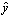— current output values of network or ensemble.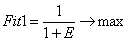(2)

where  E — value; which by formula (1) was calculated.

• The second fitness function — Complexity of network structure: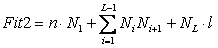(3)

where n — amount of input neurons in the first layer, Ni — amount of neurons in the i-th layer, — number of hided layer, L — the amount of hided layers in neural network, l — the amount of neurons on the last layer.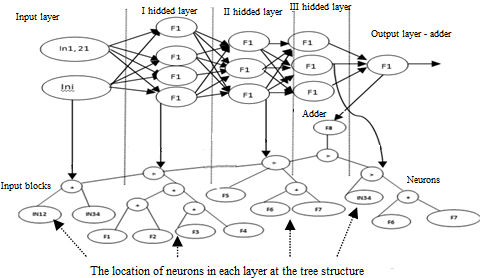Figure 1. The representation ANN into the tree structure

In evolutionary algorithms there are different types of operators and necessary to do different initial settings. To avoid choosing the algorithm settings it is reasonable to apply the self-adjusting procedure. The SelfAGP procedure works as follows:

Step 1. Initialization

Create a population of individuals — tree. Each individual is an encoded networks.

Step 2. Weight factors optimization

Optimization of the neural network weighting factors by Backpropagation. The criterion for stopping the optimization process is the minimization of the prediction error.

Step 2. Choosing evolutionary operators

In this step all combinations of EO have equal probabilities of being selected. In other step is necessary to recalculate probabilities of each combinations of EO. All combinations with different types of operators are formed. In this stage two types of selection operators (tournament, proportion), two types of mutation operators (strong, weak) and for recombination: one-point and ASR (Average Structure Recombination) «Citation» [13, p. 29] types were used. The ASR type bellow is described.

Step 3. Evaluation of criteria values

Estimation by criteria values for all individuals from the current population.

Step 4. Generation of new solutions

• Selection two individuals for recombination by VEGA (Vector Evaluated Genetic Algorithm) method «Citation» [14, p. 43]. First the VEGA method by Shafer in 1984 year is proposed. Idea of VEGA is the suitability of individuals evaluated by each of K criteria separately. Evaluated individuals in the intermediate population are collected. Amount of intermediate population for each of K criteria is , where -amount of individuals in population. Then all intermediate populations are combined to obtain a new population with size N. Then evolutionary algorithm is carried out according to the general scheme of GP algorithm.
• Recombinantion of selected individuals (parents). Recombination of selected individuals is the creation of descendents. Bellow the procedure developed for recombination — ASR; which is based on averaging the structures of the two selected individuals — ANN. The developed method is simpler for implementation and effectiveness, as the research results below are shown. Description of the ASR:
1. Determination the amount of hidden layers for the first individual — ANN: Layer1n and for the second individual: Layer2
2. Determination the amount of neurons in each hidden layer for the first individual — ANN: Neuron1n and for the second: Neuron2n, as in Tables 1 and 2 is shown: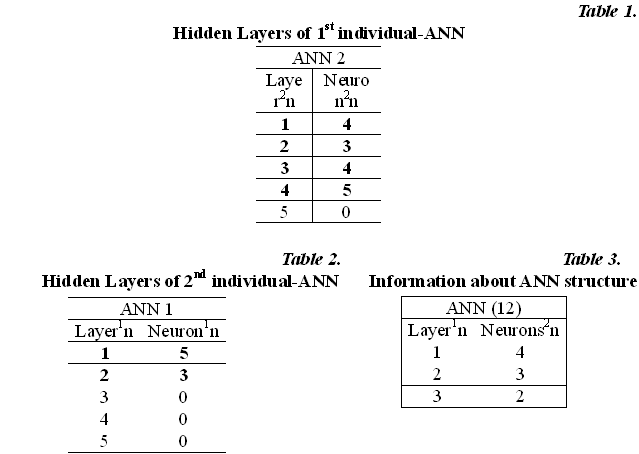1. Calculation the average amount of hidden layers (with neurons) using data; which in Table 1 and 2 is given:
1. Calculation the average amount of neurons in each hidden layer using the results of the calculations in step (c) and data from Tables 1 and 2. If the average value is not an integer — the rounding is performed. The calculation is carried out according to following formulas (5)-(7). For layer 1: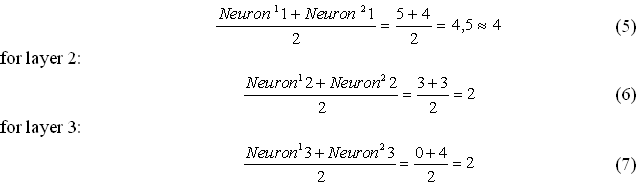1. Formation of a descendant using calculated data about the network structure as in Table 3 is shown.
2. Repetition of steps (a) — (e) for creation other descendents.
• Mutation of a descendant.
• Evaluation a descendant.
• Compilation new population (solutions) by each created descendant.

Step 5. Resources reallocation

Choose a new combination of EO by recalculation of the probability values. For recalculation need to estimate the EO combination effectiveness by formula (8) for each descendant, which was created by this EO combination: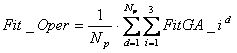(8)

where FitGA_id are fitness functions; Np is amount of descendants which were created by chosen variant of EO combination.

The number of added fitness functions may be different, it depends on the algorithm. A combination of EO with the lowest probability value changes on the “priority” variant. The recalculation of probabilities is implemented for each iteration of the algorithm. If all combinations on a «priority» option have been replaced, all probability values are cleared and self-adjusting procedure is repeated (steps 1, 5). New variants of EO combination are generated again.

Step 6. Stopping Criterion

Check the stop-criterion: if it is true, then complete the working of SelfAGP; otherwise continue from the second step. After stopping, to select the «best» individual — ANN. For K «best» ANN described procedure is carried out K times (steps 1-6).

In the second stage of SelfCGP algorithm the selection of effectiveness networks in the final ensemble (SelfAGP + ENN) is realized. The third criterion is the error of a ensemble decision. The third criteria is the prediction error of individual — ENN. The ensemble decision by Scheme ED 2 (Scheme for creation an Ensemble Decision 2) is made. The fitness function is calculated by the formula (2). The Scheme ED2 for creation an ensemble decision works as follows:

1. Calculation network output values ​​included in the ensemble using train data points.
2. Calculation the difference between the reference and real output values of network.
3. Selection network from ensemble; which a minimal difference is shown.
4. Repetition steps (a) — (c) in each train data point.
5. Determination the nearest value (point) from test and train data. Test selected in step (c) network in first test point.
6. Foundation networks as in steps (c) — (f) are shown for all test points. Calculation decision using networks; which were found in each test point. Foundation network decisions in each test points.
7. Creation total ensemble decision using network decisions, as it in step (f) is explained.

The second part of algorithm works as follows:

Step 1. Initialization

Create a population of individuals — tree. Each individual is an encoded ANN.

Step 2. Weight factors optimization

Optimization of the neural network weighting factors by Backpropagation. The criterion for stopping the optimization process is the minimization of the prediction error.

Step 3. To each generated individual the «best» individual — ANN is added. The amount of the additional «best» individual-ANNs in ensemble may be different, each of them is found by the first stage of algorithm (steps 1-12).

Step 4. Choosing evolutionary operators

In this step all combinations of EO have equal probabilities of being selected. In this stage all types of EO are used.

Step 5. Evaluation of criteria values

Estimation by criteria values for all individuals from the current population.

Step 6. Generation of new solutions

• Selection two individuals for recombination by VEGA.
• Recombinantion of selected individuals (parents). Recombination of selected individuals — the creation of descendent by the method ASR; which in detail in the first stage of algorithm was described.
• Mutation of a descendant.
• Evaluation a new descendant.
• Compilation new population (solutions) by each created descendant.

Step 7. Resources reallocation

Choose a new combination of EO by recalculation of the probability values. For recalculation need to estimate the EO combination effectiveness by formula (8) for each descendant which was created by this EO combination. New variants of EO combination are generated again as in the first stage of algorithm.

Step 8. Stopping Criterion

Check the stop-criterion: if it is true, then complete the working of SelfAGP; otherwise continue from the second step. After stopping, to select the most effectiveness K individuals for the final ensemble using VEGA.

To evaluate the efficiency of the developed algorithm several test tasks were collected. All tasks in Table 4 are represented. There are two functions for approximation (tasks 1, 2) and one » Equipment» for prediction (task 3). Task 3 for prediction from the repository UCI Machine Learning Repository «Citation» [15, p. 1] was found. In the second column in Table 4 the size of data for all tasks is represented and in the last column the number of task is determined.

Table 4.

Data base description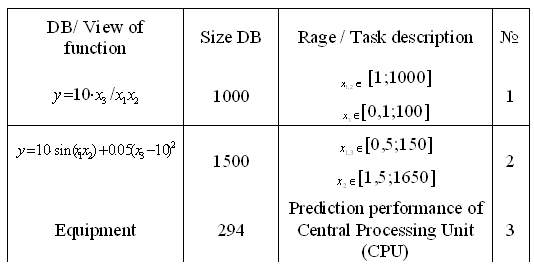For the research the software package (SP) using the object-oriented language of programming Visual Studio C # was implemented: «Software package for automated formation ensembles of intelligent information technology for solving tasks of prediction, classification and modeling using multi-objective genetic programming.» Results of the research applying SelfAGP algorithm in Tables 5, 6, 7 are presented. For the research shown in Table 5 a single ANN using first stage of SelfAGP algorithm and ENN using two stages are involved. Also the effectiveness of different types of recombination with different amount of additional «best» individual — ANNs was researched (Table 5). The efficiency of ENN decision with different amount of networks (size of ensemble) applying 2 additional «best» individual — ANNs in Table 6, 7 are shown. Also in Tables 6, 7 in first column outputs (decisions) of separate (Y1-Y8) ANN in ensemble and output (decision) of ensemble (Y(Scheme)) are shown. The following parameters were determined for the test: maximum amount of hidden layers of ANN — 5; the maximum amount of neurons in each layer of ANN — 10; the amount of activation function types — 8; amount of input neurons in ANN — 3; amount of output values in ANN — 1; amount of runs for test the SelfAGP algorithm for each type of task — 100. The data set was randomly divided into training and test samples in a proportion of 80 — 20%. The error of ensemble forecasting in percentages by the formula (9) is converted:

#####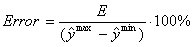(9)

where  is a value, which by formula (1) was calculated; expression (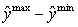) is a deference between maximum and minimum values of an ensemble decision (output values).

Table 5

##### The test results using Self AGP for all tasks with different types of recombination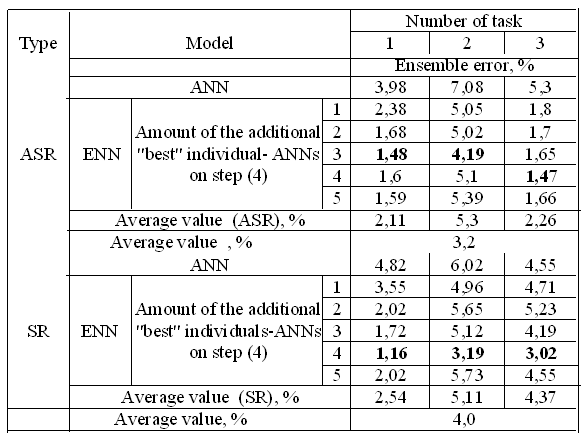Table 6.

The test results of SelfAGP for the 1st task using different amount of ANNs in ensemble

 Error, % Size of final ENN 2 3 4 5 6 7 Y1 11,8 11,9 10,0 11,5 12,5 11,3 Y2 9,1 11,1 9,0 10,7 10,3 11,3 Y3 — 11,5 11,2 11,3 12,2 11,2 Y4 — — 11,7 9,4 11,2 10,2 Y5 — — — 10,3 10,0 8,2 Y6 — — — — 9,8 11,5 Y7 — — — — — 10,8 Y(Scheme) 5,6 4,4 3,3 1,8 1,9 2,9

Table 7.

The test results of SelfAGP for the 3rd task using different amount of ANNs in ensemble

 Error, % Size of final ENN 2 3 4 5 6 7 Y1 10,4 7,8 9,2 10,2 7,9 8,5 Y2 10,6 8,3 9,5 7,6 8,8 8,7 Y3 — 8,2 8,1 9,9 9,7 10,0 Y4 — — 9,4 7,8 8,8 9,0 Y5 — — — 8,3 7,6 9,2 Y6 — — — — 10,2 8,2 Y7 — — — — — 7,3 Y(Scheme) 4,4 3,64 1,9 6,23 1,8 3,3

The results of the research applied comprehensive approach for the formation effectiveness ensembles high efficiency for all used test tasks is demonstrated. The advantage of the proposed algorithm is reducing the cost of the solution by automotive selection the most useful EO. Also proposed algorithm SelfAGP allows to use ANN with compact structure and maximum accuracy. After test can conclude, that the average modeling error in the range of 3,9 — 7,0% for single ANN and 1,1 — 4,1% for ENN (Table 5). According to the results from Table 5 the average error value for ASR type of recombination is 3,2% and for standard recombination is 4,0%. Using ASR type demonstrates lower error value than standard type. The ASR method shows the effectiveness and advisable to use this method in the further works. After the research necessary should be noted, that developed Scheme ED2 for creation total ensemble decision works with high effectiveness. As we can see the error of decisions of single ANNs in ENN and decision of total ensemble in Table 6, 7 are different. The relative difference by up to 53,5% for the 1st task and for the 3nd by up to 41,9%. After test should be noted, that the reduction of complexity of neural network models influence to the effectiveness of network and lead to increasing of prediction error. But after applying described algorithm the reduction of structure complexity is in average for neurons — 30% and for layers — 20% if to compare with initially structure complexity of network. Therefore, SelfAGP algorithm may be used in future for solving real world tasks with high efficiency. Also may be used for solving medical important tasks as creation effectiveness model of human`s state after-surgery cognitive dysfunction.

Refereneeferences:

1. Anderson, D., McNeill, G. Artificial neural networks technology. In DACS report. 1992.
2. Loseva E. D., Lipinsky L. V. Ensemble of networks with application of multi-objective self-configurable genetic programming. Vestnik SibGAU. 2016, Vol. 17, No. 1.
3. Angeline, P.J. Adaptive and self-adaptive evolutionary computations. Palaniswami M. and Attikiouzel Y. (Eds.) In Computational Intelligence: A Dynamic Systems Perspective. IEEE Press, 1995.
4. Hansen, L.K. Neural network ensembles / L.K. Hansen, P. Salamon IEEE Trans. Pattern Analysis and Machine Intelligence. 1990. 12 (10).
5. Jimenez, D. Dynamically weighted ensemble neural networks for classification / D. Jimenez in: Proc. IJCNN- 98, vol.1, Anchorage, AK. In Computer Society Press (IEEE), 1998.
6. Perrone, M.P. When networks disagree: ensemble method for neural networks / L.N. Cooper, M.P. Perrone in: R.J. Mammone (Ed.). In Artificial Neural Networks for Speech and Vision. Chapman & Hall. New York, 1993.
7. Zhi-Hua, Zhou. Ensembling Neural Networks: Many Could Be Better Than All / Wu Jianxin, Tang Wei, Zhou Zhi-Hua. In Artifitial Inteligent. 2002, vol.137, no.1-2.
8. Bukhtoyarov, V. V. The evolutionary method of forming a common solution in collectives of neural networks. In Artificial Intelligence and Decision-making. 2010, No. 3.
9. Elena Loseva, Leonid Lipinsky, Anna Kuklina. Eensembles of neural networks with application of multi-objective self-configurable genetic programming in forecasting problems. In 11th International Conference. ICNC,
10. Holger, Schwenk, Yoshua, Bengio,. Boosting Neural Networks. In Neural Computation,
11. Koza, J.R.,.Genetic Programming: On the Programming of Computers by Means of Natural Selection, MIT Press. London, 1
12. Elena Loseva, Leonid Lipinsky, Anna Kuklina. Eensembles of neural networks with application of multi-objective self-configurable genetic programming in forecasting problems. In 11th International Conference. ICNC, 2015a.
13. Rui Li, Michael T.M. Emmerich, Jeroen Eggermont, Thomas Back, M. Schutz, J. Dijkstra, J.H.C. Reiber. Mixed Evolution Strategies for Parameter Optimization. In Evolutionary Computation,
14. Ashish, G. and Satchidanada D. Evolutionary Algorithm for Multi-Criterion Optimization: A Survey. In International Journal of Computing & Information Science. 2004, vol. 2, no. 1.
15. Asuncion, D. Newman. UCI machine learning repository. University of California, Irvine, School of Information and Computer Sciences. URL: type=»book» name=»DECISION — MAKING SCHEME FOR ENSEMBLE OF NEURAL NETWORKS IN TASK OF INTELLECTUAL DATA ANALYSIS» description=»The ensemble artificial neural networks — an approach based on using several neural networks for solving the tasks. The first urgent problem is automotive modeling effectiveness neural networks is considered. For that the two-step approach for modeling neural networks using multi-criteria genetic programming (GP) and their formation in ensembles is developed. The new self-adjusting approach for GP (SelfAGP) was developed, based on choosing efficiency combination of evolutionary operators. The second urgent problem is formation general ensemble decisions by effectiveness method (Schema) is considered. For that the Scheme ED 2 is developed. Also in this paper the effectiveness of using different variants for recombination individuals was studied. All researches on different test and practical tasks were done. The obtained results showed that the developed technique provides to decrease error for prediction using ensemble decision by up to 41,9% and for approximation by up to 53,5%. The proposed technique on the tree corpora was compared. Experimental results demonstrate the effectiveness of the proposed algorithm. » author=»Loseva Elena» publisher=»Басаранович Екатерина» pubdate=»2016-12-17″ edition=»euroasia-science_28.04.2016_4(25)» ebook=»yes» ]

Список литературы:

##### euroasia
Записи созданы 9819

## МОДЕЛИРОВАНИЕ ФОТОЭЛЕКТРИЧЕСКИХ ПРЕОБРАЗОВАТЕЛЕЙ НА ОСНОВЕ НАНОГЕТЕРОСТРУКТУР GAP/SI И ALXGA1-XAS/SI (62-67)

Начните вводить, то что вы ищите выше и нажмите кнопку Enter для поиска. Нажмите кнопку ESC для отмены.

Вернуться наверх